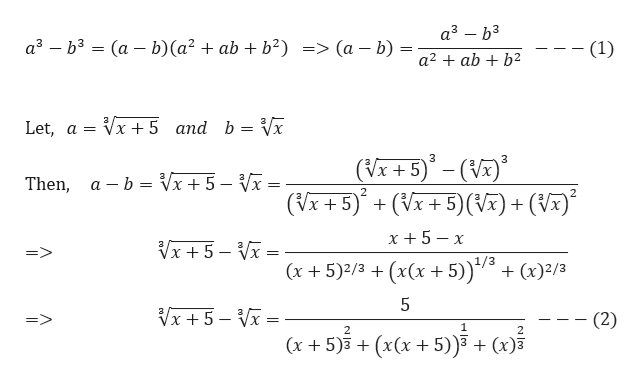# 7. Rationalize the expressionShow your work!VI + 5 - Va

Question
8 views

Practice 5.7

Question #7

check_circle

Step 1

We need to rationalize the given expression

Step 2

We know th...help_outlineImage Transcriptionclosea – b3 a – b3 = (a – b)(a² + ab + b²) => (a – b) = (1) a2 + ab + b2 Vx + 5 and b = Vx Let, a = 3 3 (Vx + 5)° – (V)* – Vĩ = a – b = Vx+ 5 Then, (Vĩ + 5)° + (Vx + 5)(V7) + (V7)° x + 5 – x Vx + 5 – Vĩ = => (x + 5)2/3 + (x(x + 5)) + (x)²/3 Vx + 5 – Vx = (2) 2 (x + 5)3 + (x(x +5))³ + (x)3 fullscreen

### Want to see the full answer?

See Solution

#### Want to see this answer and more?

Solutions are written by subject experts who are available 24/7. Questions are typically answered within 1 hour.*

See Solution
*Response times may vary by subject and question.
Tagged in

### Other